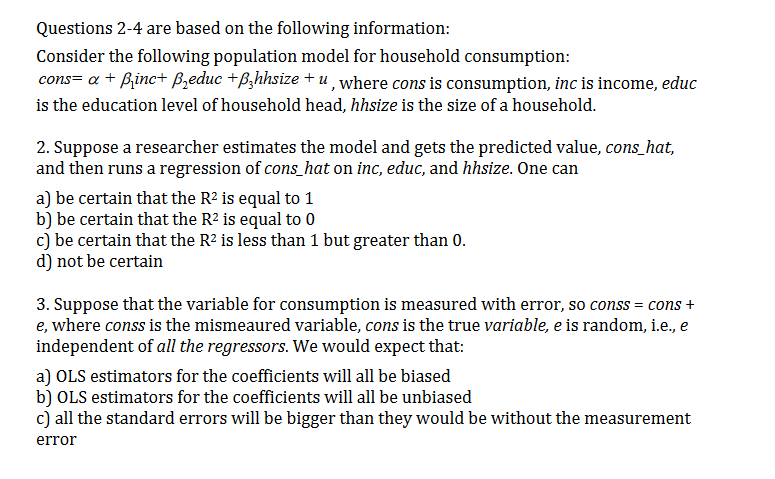# Questions 2-4 are based on the following information:Consider the following population model for household consumption:cons= a Binc+ Bzeduc +B,hhsize + u, where cons is consumption, inc is income, educis the education level of household head, hhsize is the size of a household.2. Suppose a researcher estimates the model and gets the predicted value, cons_hat,and then runs a regression of cons_hat on inc, educ, and hhsize. One cana) be certain that the R2 is equal to 1b) be certain that the R2 is equal to 0c) be certain that the R2 is less than 1 but greater than 0.d) not be certain3. Suppose that the variable for consumption is measured with error, so conss = cons +e, where conss is the mismeaured variable, cons is the true variable, e is random, i.e., eindependent of all the regressors. We would expect that:a) OLS estimators for the coefficients will all be biasedb) OLS estimators for the coefficients will all be unbiasedc) all the standard errors will be bigger than they would be without the measurementerror

Question
314 views

#2help_outlineImage TranscriptioncloseQuestions 2-4 are based on the following information: Consider the following population model for household consumption: cons= a Binc+ Bzeduc +B,hhsize + u, where cons is consumption, inc is income, educ is the education level of household head, hhsize is the size of a household. 2. Suppose a researcher estimates the model and gets the predicted value, cons_hat, and then runs a regression of cons_hat on inc, educ, and hhsize. One can a) be certain that the R2 is equal to 1 b) be certain that the R2 is equal to 0 c) be certain that the R2 is less than 1 but greater than 0. d) not be certain 3. Suppose that the variable for consumption is measured with error, so conss = cons + e, where conss is the mismeaured variable, cons is the true variable, e is random, i.e., e independent of all the regressors. We would expect that: a) OLS estimators for the coefficients will all be biased b) OLS estimators for the coefficients will all be unbiased c) all the standard errors will be bigger than they would be without the measurement error fullscreen
check_circle

star
star
star
star
star
1 Rating
Step 1

2)

If the no of independent variable increases, Coefficient of determination i.e. R2 increases and  R2 value is...

### Want to see the full answer?

See Solution

#### Want to see this answer and more?

Solutions are written by subject experts who are available 24/7. Questions are typically answered within 1 hour.*

See Solution
*Response times may vary by subject and question.
Tagged in

### Other